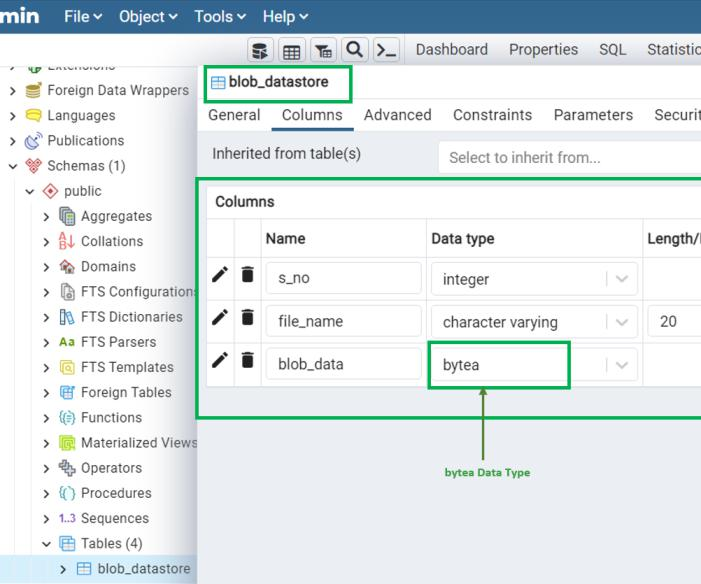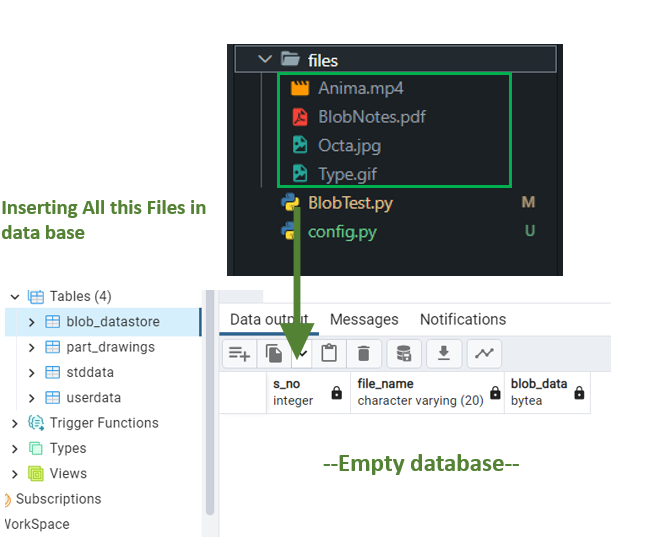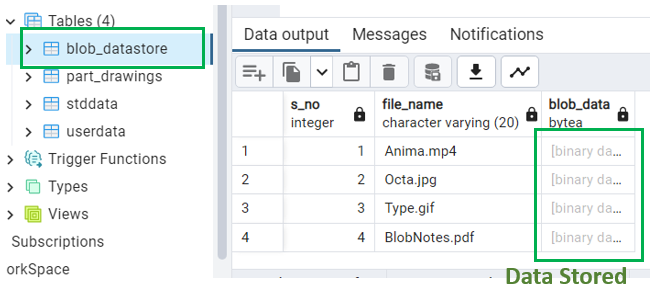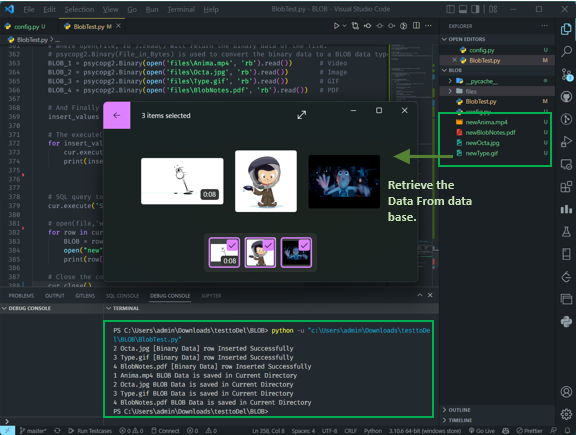Open in App
Not now

# Handling PostgreSQL BLOB data in Python

• Last Updated : 21 Nov, 2022

In this article, we will learn how to Handle PostgreSQL BLOB data in Python.

• BLOB is a Binary large object (BLOB) is a data type that can store any binary data.
• To store BLOB data in a PostgreSQL database, we need to use the Binary Large Object (BLOB) data type.
• By using the Binary Large Object (BLOB) data type, we can store any binary data in a PostgreSQL database.

The different types of BLOB data that we can store in the Database are [Extension]:

• .png
• .jpg
• .gif
• .pdf
• .docx
• .xlsx
• .mp4, .mp3 …etc.

psycopg2 is a Python module that allows us to connect to a PostgreSQL database & Store BLOB Data.

### BLOB Datatype

A BLOB (binary large object) is a varying-length binary string. And that string will be stored in the database as a bytea(Byte Array) Datatype.
The table for storing BLOB data in PostgreSQL is called as Large Object table and the data type is bytea.

`psycopg2.Binary(File_in_Bytes)`

## Handling PostgreSQL BLOB data in Python

### Read & Write Operation in Binary:

To read or write a file (like an image) in binary we can use the open() function. Where the ‘rb’ is for reading binary And the ‘wb’ is for writing binary.

```open(FileName, 'rb').read()  # 'rb' stands for Read Binary
open(FileName, 'wb').write(BLOB) # 'wb' stands for Write Binary```

### Table to Store BLOB:

Let’s the table name be blob_datastore & The Datatype’s:

• For Storing the Name of the file we will use Character varying Data type
• To Store the BLOB datatype we are going to use the bytea datatype.Handling PostgreSQL BLOB data

### The Complete Function to insert the BLOB data into the database.

Here we are inserting the BLOB data into the database after establishing the connection with database using the .execute() function we are inserting the required values.

## Python3

 `import` `psycopg2``from` `config ``import` `config`` ` `# This Function will open & convert the image or file data to binary data.`` ` ` ` `def` `convert_To_Binary(filename):``    ``with ``open``(filename, ``'rb'``) as ``file``:``        ``data ``=` `file``.read()``    ``return` `data`` ` ` ` `def` `insert_BLOB(S_No, FileName):``    ``""" insert a BLOB into a table """``    ``conn ``=` `None``    ``try``:`` ` `        ``# connect to the PostgreSQL server & creating a cursor object``        ``conn ``=` `psycopg2.connect(``*``*``config)`` ` `        ``# Creating a cursor with name cur.``        ``cur ``=` `conn.cursor()`` ` `        ``# Binary Data``        ``file_data ``=` `convert_To_Binary(FileName)`` ` `        ``# BLOB DataType``        ``BLOB ``=` `psycopg2.Binary(file_data)`` ` `        ``# SQL query to insert data into the database.``        ``cur.execute(``            ``"INSERT INTO blob_datastore(s_no,file_name,blob_data) VALUES(%s,%s,%s)"``, (S_No, FileName, BLOB))`` ` `        ``# Close the connection``        ``cur.close()`` ` `    ``except``(Exception, psycopg2.DatabaseError) as error:``        ``print``(error)``    ``finally``:``        ``if` `conn ``is` `not` `None``:``            ``# Commit the changes to the database``            ``conn.commit()`` ` ` ` `insert_BLOB(``1``, ``'newOcta.jpg'``)`

### The Complete Function to Retrieve the BLOB data into the database.

Here we are fetching the BLOB data from the database after establishing the connection with database using the .execute() function we are fetching all the required values using the fetchall() function.

## Python3

 `# Complete Function to Retrieve the BLOB data into the database.``import` `psycopg2``from` `config ``import` `config`` ` `# This Function will Creates File from binary data.`` ` ` ` `def` `Binary_To_File(BLOB, FileName, oldFileName):``    ``with ``open``(f``"{FileName}"``, ``'wb'``) as ``file``:``        ``file``.write(BLOB)``    ``print``(f``"{oldFileName} File saved With Name name {FileName}"``)`` ` ` ` `def` `retrieve_BLOB(S_No, newFileName):``    ``""" Retrieve a BLOB From a table """``    ``conn ``=` `None``    ``try``:``        ``# connect to the PostgreSQL server & creating a cursor object``        ``conn ``=` `psycopg2.connect(``*``*``config)`` ` `        ``# Creating a cursor with name cur.``        ``cur ``=` `conn.cursor()`` ` `        ``# Retrieve BLOB data from the database.``        ``cur.execute(``'SELECT * FROM BLOB_DataStore'``)``        ``db ``=` `cur.fetchall()`` ` `        ``BLOB ``=` `db[S_No``-``1``][``2``]``        ``# open("FromDB"+db, 'wb').write(BLOB)``        ``Binary_To_File(BLOB, newFileName, db[S_No``-``1``][``1``])`` ` `        ``# Close the connection``        ``cur.close()`` ` `    ``except``(Exception, psycopg2.DatabaseError) as error:``        ``print``(error)``    ``finally``:``        ``if` `conn ``is` `not` `None``:``            ``# Commit the changes to the database``            ``conn.commit()`` ` ` ` `retrieve_BLOB(``1``, ``'OctaFromDB.jpg'``)`

Example:

Storing All the Files(Anima.mp4, BlobNotes.pdf, Octa.jpg, Type.gif) in the Table named blob_datastore And retrieving The table data.Handling PostgreSQL BLOB data

Code to insert BLOB data:

Here we are basically establishing the connection with the database, then creating the cursor and creating the table and inserting the values according to its data type i.e. varchar, bytea, etc. finally reading the large file into its binary form and storing in the database.

## Python3

 `import` `psycopg2`` ` `conn ``=` `None``try``:``    ``# connect to the PostgreSQL server``    ``conn ``=` `psycopg2.connect(``        ``host``=``'localhost'``,``        ``dbname``=``'For_Practice'``,``        ``user``=``'postgres'``,``        ``password``=``'321654'``,``        ``port``=``5432``    ``)`` ` `    ``# Creating a cursor with name cur.``    ``cur ``=` `conn.cursor()``    ``cur.execute(``        ``"CREATE TABLE blob_datastore (s_no serial, file_name VARCHAR ( 50 ), blob_data bytea)"``)``    ``# SQL query to insert data into the database.``    ``insert_script ``=` `'''``        ``INSERT INTO blob_datastore(s_no,file_name,blob_data) VALUES (%s,%s,%s);``    ``'''`` ` `    ``# open('File,'rb').read() is used to read the file.``    ``# where open(File,'rb').read() will return the binary data of the file.``    ``# psycopg2.Binary(File_in_Bytes) is used to convert the binary data to a BLOB data type.``    ``BLOB_1 ``=` `psycopg2.Binary(``        ``open``(``'files\Anima.mp4'``, ``'rb'``).read())       ``# Video``    ``BLOB_2 ``=` `psycopg2.Binary(``        ``open``(``'files\Octa.jpg'``, ``'rb'``).read())        ``# Image``    ``BLOB_3 ``=` `psycopg2.Binary(``open``(``'files\Type.gif'``, ``'rb'``).read())        ``# GIF``    ``BLOB_4 ``=` `psycopg2.Binary(``open``(``'files\BlobNotes.pdf'``, ``'rb'``).read())   ``# PDF`` ` `    ``# And Finally we pass the above mentioned values to the insert_script variable.``    ``insert_values ``=` `[(``1``, ``'Anima.mp4'``, BLOB_1), (``2``, ``'Octa.jpg'``, BLOB_2),``                     ``(``3``, ``'Type.gif'``, BLOB_3), (``4``, ``'BlobNotes.pdf'``, BLOB_4)]`` ` `    ``# The execute() method with the insert_script & insert_value as argument.``    ``for` `insert_value ``in` `insert_values:``        ``cur.execute(insert_script, insert_value)``        ``print``(insert_value[``0``], insert_value[``1``],``              ``"[Binary Data]"``, ``"row Inserted Successfully"``)`` ` `    ``# SQL query to fetch data from the database.``    ``cur.execute(``'SELECT * FROM BLOB_DataStore'``)`` ` `    ``# open(file,'wb').write() is used to write the binary data to the file.``    ``for` `row ``in` `cur.fetchall():``        ``BLOB ``=` `row[``2``]``        ``open``(``"new"``+``row[``1``], ``'wb'``).write(BLOB)``        ``print``(row[``0``], row[``1``], ``"BLOB Data is saved in Current Directory"``)`` ` `    ``# Close the connection``    ``cur.close()`` ` `except``(Exception, psycopg2.DatabaseError) as error:``    ``print``(error)``finally``:``    ``if` `conn ``is` `not` `None``:``       ` `        ``# Commit the changes to the database``        ``conn.commit()`

Output:

Data Storing:Handling PostgreSQL BLOB data

Data Retrieving:Handling PostgreSQL BLOB data

My Personal Notes arrow_drop_up